×#### Thank you for registering.

One of our academic counsellors will contact you within 1 working day.

Click to Chat

1800-1023-196

+91-120-4616500

CART 0

• 0

MY CART (5)

Use Coupon: CART20 and get 20% off on all online Study Material

ITEM
DETAILS
MRP
DISCOUNT
FINAL PRICE
Total Price: Rs.

There are no items in this cart.
Continue Shopping```Geometrical Representation of Fundamental OperationsThe various fundamental laws can be represented graphically. The graphical representation makes it easier to understand the laws. The four fundamental laws of geometry have been explained below:If two points P and Q represent complex numbers z1 and z2 respectively in the Argand Plane, then the sum z1+z2 is represented by the extremity R of the diagonal OR of parallelogram OPRQ having OP and OQ as two adjacent sides. The addition of the complex numbers z1 and z2 can be assumed to be the addition of the vectors by utilizing the parallelogram law.

(ii) Geometrical representation of subtraction:

The representation of the difference of two complex numbers is slightly complicated than the addition of the complex numbers. The easiest way of presenting the subtraction is to think of addition of a negative vector. If we want to represent z1-z2, then the easiest way of representing it would be to think of adding a negative vector z1 + (-z2). The negative vector is the same as the positive one, the only difference being that the negative vector points in the opposite direction.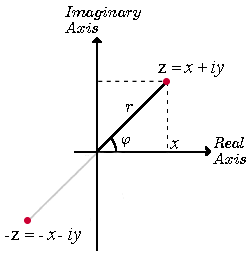The difference vector z1 - z2 is represented below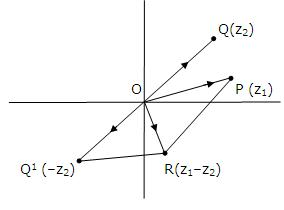Remark: It is important to note here that the vector representing the difference of the vectors z1 - z2 may also be drawn joining the end point of z2 to the tip of z1 instead of the origin. This kind of representation does not alter the meaning or interpretation of the difference operator. The difference operator joining the tips of z1 and z2 is represented below: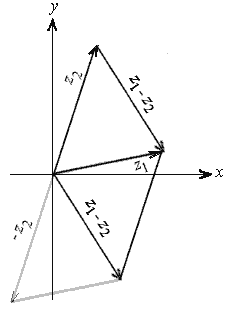(iii) Modulus and argument of multiplication of two complex numbers: Modulus and argument are two extremely important concepts associated with complex numbers.

Modulus of a complex number: The modulus of a complex number is defined to be the distance of the number from the origin. It is denoted by |z| and the value of the modulus of z = x+iy is √x2+y2.

Argument of a complex Number: Argument of a complex number is basically the angle that explains the direction of the complex number. It is measured in radians. If we have the complex number in polar form i.e. z = r(cos θ + i sin θ), then its argument is θ.

Modulus of product of two complex numbers: Let z1 and z2 be two complex numbers in polar form

z1 = r1(cos θ1 + i sin θ1)

z2 = r2(cos θ2 + i sin θ2)

Hence their product is given by

z1z2 = r1r2 [(cos θ1 cos θ2 – sin θ1 sin θ2) + i(sin θ1 cos θ2 + cos θ1 sin θ2)].

This expression can be further simplified as

z1z2 = r1r2 [(cos (θ1 + θ2) + i sin (θ1 + θ2)].

The product z1z2 has the modulus r1r2 and the argument (θ1 + θ2).

Theorem: For any two complex numbers z1, z2

We have, |z1, z2| = |z1| |z2| and arg(z1, z2) = arg(z1) + arg(z2)

Proof: z1 = r1 eiθ1, z2 = r2 eiθ2

z1z2 = r1 r2 ei(θ1+θ2)

=> |z1 z2|=|z1|=|z2|

arg (z1z2) = arg(z1) + arg(z2)

i.e., to multiply two complex numbers, we multiply their absolute values and add their arguments.

View the following video for more on argument and modulus

Some Key Points

(i) P.V. arg(z1z2) ≠ P.V. arg(z1) + P.V. arg(z2)

(ii) |z1z2 ...... zn| = |z1| |z2| ......... |zn|

(iii) arg(z1.z2 ......... zn) = argz1+argz2+ ........ + argzn

(iv) Geometrical representation of multiplication of complex numbers -

Let P, Q be represented by z1= r1 eiθ1, z2 = r2 eiθ2 respectively. To find point R representing complex number z1 z2, we take a point L on real axis such that OL=1 and draw triangle OQR similar to triangle OLP. Therefore,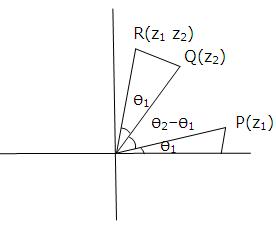OR/OQ = OP/OL = OR = OP*OQ

i.e., OR = r1 r2 and ∠ QOR = θ,

R(z1z2) Q(z2) P(z1)

∠ LOR = ∠ LOP + ∠ POQ + ∠ QOR

= Q1 + Q2 - Q1 + Q1

= Q1 + Q2

Hence, R is represented by z1 z2 = r1 r2 ei(Q1+Q2)

(v) Modulus and argument of division of two complex numbers:

Theorem: If z1 and z2 ( ≠0 ) are two complex numbers, then |z1/z2| =

|z1|/|z2|   and arg(z1/z2) = arg z1 - arg z2

Note:

P.V. arg(z1/z2) ≠  P.V. arg (z1) - P.V. arg (z2)

(vi) Geometrical representation of the division of complex numbers-

Let P, Q be represented by z1= r1 eiθ1, z2 = r2 eiθ2 respectively. To find point R representing complex number z1/z2, we tale a point L on real axis such that OL=1 and draw a triangle OPR similar to OQL.

Therefore, OP/OQ = OR/OL

=> OR = r1/r2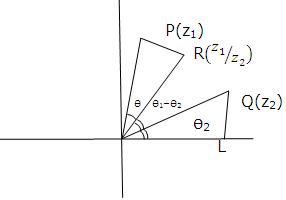and ∠LOR = ∠LOP - ∠ROP

= θ1 - θ2

Hence, R is represented by z1/z2 =r1/r2 ei(θ1 - θ2)

askIITians offers comprehensive study material which covers all the topics of IIT JEE Mathematics syllabus in detail. It is important to have a strong grip on the various algebraic operators as they lay the foundation of several other topics. The JEE aspirants must master the topic in order to remain competitive in the JEE.

Related Resources

Look into the Previous Year Papers with Solutions to get a hint of the kinds of questions asked in the exam.

You can get the knowledge of Useful Books of Mathematics here.

To read more, Buy study materials of Complex Numbers comprising study notes, revision notes, video lectures, previous year solved questions etc. Also browse for more study materials on Mathematics here.
```### Course Features

• 731 Video Lectures
• Revision Notes
• Previous Year Papers
• Mind Map
• Study Planner
• NCERT Solutions
• Discussion Forum
• Test paper with Video Solution